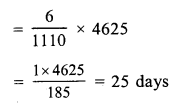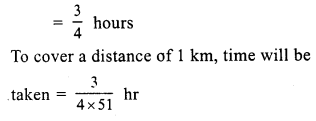# RS Aggarwal Class 7 Solutions Chapter 9 Unitary Method Ex 9A

In this chapter, we provide RS Aggarwal Solutions for Class 7 Chapter 9 Unitary Method Ex 9A for English medium students, Which will very helpful for every student in their exams. Students can download the latest RS Aggarwal Solutions for Class 7 Chapter 9 Unitary Method Ex 9A Maths pdf, free RS Aggarwal Solutions Class 7 Chapter 9 Unitary Method Ex 9A Maths book pdf download. Now you will get step by step solution to each question.

### RS Aggarwal Solutions for Class 7 Chapter 9 Unitary Method Ex 9A Download PDF

Question 1.
Solution:
Cost of 15 oranges = Rs. 110
Cost of 1 orange = Rs. 11015
and cost of 39 oranges = Rs. 11015 x 39
= Rs. 22 x 13 = Rs. 286

Question 2.
Solution:
In Rs. 260, the sugar is bought = 8 kg
and in Re. 1, the sugar is bought = 8260 kg
Then in Rs. 877.50, the sugar will be bought = 8260 x 877.50 kg
= 8260 x 87750100
= 27 kg

Question 3.
Solution:
In Rs. 6290, silk is purchased = 37 m
and in Re. 1, silk is purchased = 376290 m
and in Rs. 4420, silk will be purchased 37
= 376290 x 4420 m = 26 m

Question 4.
Solution:
Rs. 1110 is wages for = 6 days.
Re. 1 will be wages for = 61110 days
and Rs. 4625 will be wages forQuestion 5.
Solution:
In 42 litres of petrol, a car covers = 357 km
and in 1 litre, car will cover = 35742 km
and in 12 litres, car will cover = 35742 x 12 = 102 km

Question 6.
Solution:
Cost of travelling 900 km is = Rs. 2520
and cost of 1 km will be = Rs. 2520900
andcostof360kmwillbe = Rs. 2520900 x 360 = Rs. 1008

Question 7.
Solution:
To cover a distance of 51 km, time is taken = 45 minutesQuestion 8.
Solution:
If weight is 85.5 kg, then length of iron rod = 22.5 m
If weight is 1 kg, then length of rod will beQuestion 9.
Solution:
In 162 grams, sheets are = 6Question 10.
Solution:
1152 bars of soap can be packed in 8 cartons
1 bar of soap coil be packed inQuestion 11.
Solution:
In 44 mm of thickness, cardboards are = 16
In 1 mm of thickness, cardboards will beQuestion 12.
Solution:
If length of shadow is 8.2 m, then
height of flag staff is = 7 m
If length of shadow is 1 m, then height willQuestion 13.
Solution:
16.25 m long wall is build by = 15 menQuestion 14.
Solution:
1350 litres of milk cm be consumed by = 60 patients
1 litres of milk can be consumed by = 601350 patients
and 1710 litres of milk can be consumedQuestion 15.
Solution:
2.8 cm extension is produced by = 150 g.
1 cm extension will be produced by = 1502.8 g
and 19.6 cm extension will be produced byAll Chapter RS Aggarwal Solutions For Class 7 Maths

—————————————————————————–

All Subject NCERT Exemplar Problems Solutions For Class 7

All Subject NCERT Solutions For Class 7

*************************************************

I think you got complete solutions for this chapter. If You have any queries regarding this chapter, please comment on the below section our subject teacher will answer you. We tried our best to give complete solutions so you got good marks in your exam.

If these solutions have helped you, you can also share rsaggarwalsolutions.in to your friends.